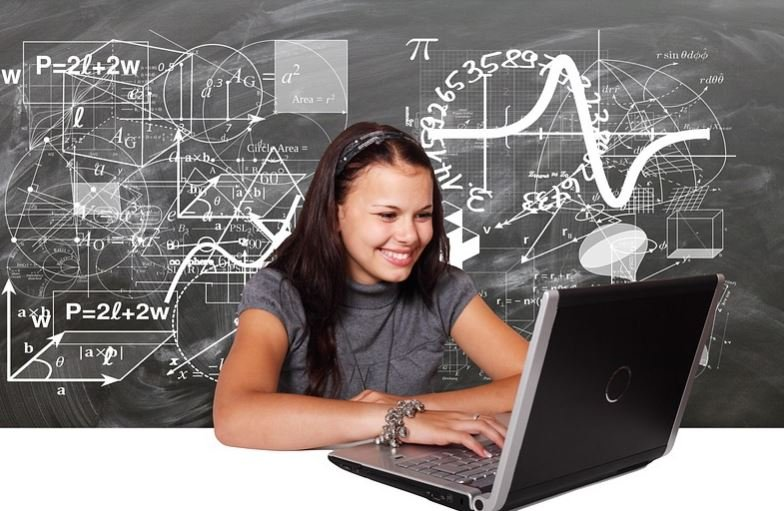Best Tips to Learn Physics Efficiently

Physics is one of the most practical subjects. The subject deals with the nature, its phenomena and the interaction of matter and its constituents.

It includes several theories, laws and relations. Students who get engaged with the concepts and relate the concepts practically start loving the subject and learn the topics in an effective way.Photo Credit

1] Give Importance to the Basics

Physics is full of theories, laws and formulas. It is extremely important to understand some of the basic concepts to be able to understand most of the other concepts.

Topics like Newton’s laws of motions, Ohm’s law, Faraday’s law, etc. are very fundamental but several other concepts revolve around these laws.

2] Draw Diagrams

Drawing diagrams can help to simplify a lot of complicated problems. For example, in mechanics, any problem becomes easy if the free body diagram is drawn. Similarly, for questions related to electricity and circuits, always draw circuit diagrams.

3] Understand the Derivations

It is always suggested to understand the derivations rather than just memorizing them. By understanding the derivations, one can have an in-depth understanding of the concepts which can help to comprehend higher level concepts more effectively.

4] Visualize

Visualization can help a lot to learn physics in a more efficient way. With visualization, most of the complex problems become easy.

Topics like, electric motors, electromagnetic radiation, waves, mechanics, Newton’s laws of motion can be understood in a better way if visualized. By visualizing, not only understanding improves but also memory retention increases.

5] Develop Maths Skills

Many topics in physics include several mathematical elements. One needs a proper mathematical foundation to be able to comprehend certain topics and solve physics questions efficiently. Maths topics especially, trigonometry and calculus are extremely important for several physics topics.

6] Relate the Concepts Practically

It is known that physics is a very practical subject and that most of its concepts can be related practically. By relating practically, understanding the concepts becomes better and engagement increases. This eventually leads to more interest and better memory retention.

These were a few tips that can help the students to learn physics in a more efficient way. Physics, if understood properly, can be extremely interesting. It is important to question the rationale of nature and relate which physics law or theory is governing them.

Students are also suggested to subscribe to BYJU’S YouTube channel where a lot of physics topics are explained in a very easy, engaging and efficient way.